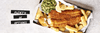Get in touch and let us know how we can help. Get in touch and let us know how we can help. Get in touch and let us know how we can help. Get in touch and let us know how we can help. Get in touch and let us know how we can help. Get in touch and let us know how we can help. Get in touch and let us know how we can help. Get in touch and let us know how we can help. Get in touch and let us know how we can help. Get in touch and let us know how we can help. Get in touch and let us know how we can help. Get in touch and let us know how we can help. Get in touch and let us know how we can help. Get in touch and let us know how we can help. Get in touch and let us know how we can help. Get in touch and let us know how we can help. Get in touch and let us know how we can help.